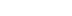/

In-depth: Floating-point complexities

In this reprinted #altdevblogaday in-depth piece, Valve Software programmer Bruce Dawson shares a list of useful -- and sometimes surprising -- facts about floating-point math.
[In this reprinted #altdevblogaday in-depth piece, Valve Software programmer Bruce Dawson shares a list of useful -- and sometimes surprising -- facts about floating-point math.] Binary floating-point math is complex and subtle. I've collected here a few of my favorite oddball facts about floating-point math, based on the articles so far in my floating-point series. The focus in this list is on float, but the same concepts all apply to double. These oddities don't make floating-point math bad, and in many cases these oddities can be ignored. But when you try to simulate the infinite expanse of the real-number line with 32-bit or 64-bit numbers, then there will inevitably be places where the abstraction breaks down, and it's good to know about them. Some of these facts are useful, and some of them are surprising. You get to decide which is which.
• Adjacent floats (of the same sign) have adjacent integer representations, which makes generating the next (or all) floats trivial
• FLT_MIN is not the smallest positive float (FLT_MIN is the smallest positive normalized float)
• The smallest positive float is 8,388,608 times smaller than FLT_MIN
• FLT_MAX is not the largest positive float (it's the largest finite float, but the special value infinity is larger)
• 0.1 cannot be exactly represented in a float
• All floats can be exactly represented in decimal
• Over a hundred decimal digits of mantissa are required to exactly show the value of some floats
• 9 decimal digits of mantissa (plus sign and exponent) are sufficient to uniquely identify any float
• The Visual C++ debugger displays floats with 8 mantissa digits
• The integer representation of a float is a piecewise linear approximation of the base-2 logarithm of that float
• You can calculate the base-2 log of an integer by assigning it to a float
• Most float math gives inexact results due to rounding
• The basic IEEE math operations guarantee perfect rounding
• Subtraction of floats with similar values (f2 * 0.5 <= f1 <= f2 * 2.0) gives an exact result, with no rounding
• Subtraction of floats with similar values can result in a loss of virtually all significant figures (even if the result is exact)
• Minor rearrangements in a calculation can take it from catastrophic cancellation to 100% accurate
• Storing elapsed game time in a float is a bad idea
• Comparing floats requires care, especially around zero
• sin(float(pi)) calculates a very accurate approximation to pi-float(pi)
• From 2^24 to 2^31, an int32_t has more precision than a float – in that range an int32_t can hold every value that a float can hold, and millions more
• pow(2.0f, -149) should calculate the smallest denormal float, but with VC++ it generates zero. pow(0.5f, 149) works.
• IEEE float arithmetic guarantees that "if (x != y) return z / (x-y);" will never cause a divide by zero, but this guarantee only applies if denormals are supported
• Denormals have horrible performance on some older hardware, which leads to some developers disabling them
• If x is a floating-point number then "x == x" may return false – if x is a NaN
• Calculations done with higher-precision intermediate values sometimes give more accurate results, sometimes less accurate results, and sometimes just inconsistent results
• Double rounding can lead to inaccurate results, even when doing something as simple as assigning a constant to a float
• You can printf and scanf every positive float in less than fifteen minutes
Do you know of some other surprising or useful aspects of floats? Respond in the comments. [This piece was reprinted from #AltDevBlogADay, a shared blog initiative started by @mike_acton devoted to giving game developers of all disciplines a place to motivate each other to write regularly about their personal game development passions.]

Latest Jobs

Pocketwatch Games

Remote
01.05.22
Senior Gameplay Engineer

Disbelief

Cambridge, Massachusetts
01.07.22
Jr. Programmer

Gameloft Australia

Brisbane, Australia
01.06.21
Creative Director

Sucker Punch Productions

Bellevue, Washington
01.06.22
Senior Systems Designer
More Jobs